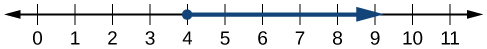## Using Interval Notation

Indicating the solution to an inequality such as $x\ge 4$ can be achieved in several ways.

We can use a number line as shown in Figure 2. The blue ray begins at $x=4$ and, as indicated by the arrowhead, continues to infinity, which illustrates that the solution set includes all real numbers greater than or equal to 4.Figure 2

We can use set-builder notation: $\{x|x\ge 4\}$, which translates to “all real numbers x such that x is greater than or equal to 4.” Notice that braces are used to indicate a set.

The third method is interval notation, in which solution sets are indicated with parentheses or brackets. The solutions to $x\ge 4$ are represented as $\left[4,\infty \right)$. This is perhaps the most useful method, as it applies to concepts studied later in this course and to other higher-level math courses.

The main concept to remember is that parentheses represent solutions greater or less than the number, and brackets represent solutions that are greater than or equal to or less than or equal to the number. Use parentheses to represent infinity or negative infinity, since positive and negative infinity are not numbers in the usual sense of the word and, therefore, cannot be “equaled.” A few examples of an interval, or a set of numbers in which a solution falls, are $\left[-2,6\right)$, or all numbers between $-2$ and $6$, including $-2$, but not including $6$; $\left(-1,0\right)$, all real numbers between, but not including $-1$ and $0$; and $\left(-\infty ,1\right]$, all real numbers less than and including $1$. The table below outlines the possibilities.

Set Indicated Set-Builder Notation Interval Notation
All real numbers between a and b, but not including a or b $\{x|a<x<b\}$ $\left(a,b\right)$
All real numbers greater than a, but not including a $\{x|x>a\}$ $\left(a,\infty \right)$
All real numbers less than b, but not including b $\{x|x<b\}$ $\left(-\infty ,b\right)$
All real numbers greater than a, including a $\{x|x\ge a\}$ $\left[a,\infty \right)$
All real numbers less than b, including b $\{x|x\le b\}$ $\left(-\infty ,b\right]$
All real numbers between a and b, including a $\{x|a\le x<b\}$ $\left[a,b\right)$
All real numbers between a and b, including b $\{x|a<x\le b\}$ $\left(a,b\right]$
All real numbers between a and b, including a and b $\{x|a\le x\le b\}$ $\left[a,b\right]$
All real numbers less than a or greater than b $\{x|x<a\text{ and }x>b\}$ $\left(-\infty ,a\right)\cup \left(b,\infty \right)$
All real numbers $\{x|x\text{ is all real numbers}\}$ $\left(-\infty ,\infty \right)$

### Example 1: Using Interval Notation to Express All Real Numbers Greater Than or Equal to a

Use interval notation to indicate all real numbers greater than or equal to $-2$.

### Solution

Use a bracket on the left of $-2$ and parentheses after infinity: $\left[-2,\infty \right)$. The bracket indicates that $-2$ is included in the set with all real numbers greater than $-2$ to infinity.

### Try It 1

Use interval notation to indicate all real numbers between and including $-3$ and $5$.

Solution

### Example 2: Using Interval Notation to Express All Real Numbers Less Than or Equal to a or Greater Than or Equal to b

Write the interval expressing all real numbers less than or equal to $-1$ or greater than or equal to $1$.

### Solution

We have to write two intervals for this example. The first interval must indicate all real numbers less than or equal to 1. So, this interval begins at $-\infty$ and ends at $-1$, which is written as $\left(-\infty ,-1\right]$.

The second interval must show all real numbers greater than or equal to $1$, which is written as $\left[1,\infty \right)$. However, we want to combine these two sets. We accomplish this by inserting the union symbol, $\cup$, between the two intervals.

$\left(-\infty ,-1\right]\cup \left[1,\infty \right)$

### Try It 2

Express all real numbers less than $-2$ or greater than or equal to 3 in interval notation.

Solution